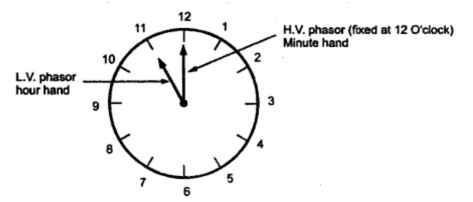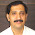### Three Phase Transformer Phasor Groups

Three phase transformer can be connected in variety of different ways such as in star, delta, V or zigzag. In many cases, vector diagrams of the primary and secondary e.m.f.s are useful to describe the characteristics, advantages and disadvantages of a given type of connection. A vector diagram can be constructed based on following principles,
1. The voltage of primary and secondary windings on the same limb are in phase opposition and the two induced e.m.f.s are in phase.
2. The e.m.f.s induced in the three phases are equal, balanced and displaced mutually by one third period in time and have a definite sequence.
In polyphase transformers, polarity alone is sufficient to represent a definite relation between the high voltage and low voltage windings. Thus we make use of vector diagrams in addition to lead markings to represent angular phase displacement between the high voltage and low voltage windings and the time order of phase sequence.
It can be seen that all phases in case of a three phase transformer have the same relative polarity which is expressed in terms of single phase polarity. If polarity of one of the phase is additive then the polarity of other two phases will additive while if polarity of one of the phases is subtractive then the polarity of other two phases will also be additive.
1.1 Nomenciature of Transformer Phasor Groups
According to B.S. (British Standard), transformer terminals are brought out in rows with high voltage windingon one side while low voltage winding on the other side. They are lettered from left to right facing the h.v. side. The h.v. terminals are represented with capital letters e.g. A, B, C and l.v. terminals are represented with small letter e.g. a, b, c. If transformer has tirtary winding, then it is represented in capital letters enclosed in circles.
The neutral terminals are represented after line terminals. The two ends of each winding are designated by subscript numbers 1, 2. If there are intermediary tappings these are numbered in order of their separation from end 1 e.g. if h.v. winding on phase B has three tappings then it would be represented as B1, B2, B3, B3 , B4 , B5 with B1 and B5 forming the phase terminals.
If the voltage induced in h.v. phase B1 B2 is in the direction of B1 to B2 at a given instant then the induced e.m.f. in the corresponding l.v. phase at the same instant will be from b1 to b2. The polyphase transformers are given some symbols to show the type of phase connection and the angle of advance turned through in passing from the vector representing the h.v. e.m.f. to that representing the l.v. e.m.f. at the corresponding terminal.
The angle of advance can be represented by a clockface hour figure. The h.v. side phasor is considered as minute hand which is always set at 12 O'clock (zero) position and the corresponding l.v. side phasor is shown with the hour hand.
For example, if the connection is written as ''Dy 11'' then it represent h.v. side delta connected while l.v. side star connected 3 phase transformer. The l.v. e.m.f. vector in a given phase combination is at 11 O'clock position i.e. + 30o in advance of the 12 O'clock position of the h.v. e.m.f. position. This is represented in the Fig. 1.Fig. 1

The groups into which all possible three phase transformer connections are classified are given below :
Group 1 : Zero phase displacement (Yy0, Dd0, Dz0)
Group 2 : 180o phase displacement (Yy6, Dd6, Dz6)
Group 3 : 30o lag phase displacement (Dy1, Yd1, Yz1)
Group 4 : 30o lead phase displacement ( Dy11, Yd11, Yz11)
The vector diagram for different types of connections is tabulated in the Table 1. The star-star connection is most economical for small, high voltage transformers. The number of turns per phase and insulation required is less. The star point can be used in case of three phase four wire system. The third harmonic voltage and current is absent in such type of connection with three phase wire system. or shell type of three phase units, the third harmonic phase voltage may be high. This type of connection is more suitable for core type transformers.
Refer the Table 1.
The delta-delta connection is more suitable for large, low voltage transformers. It increases number of turns per phase and reduces the necessary cross sectional area of conductors thus insulation problem is not present. It gives satisfactory performance even if there is large unbalance in load. Due to closed delta, third harmonic voltages are absent. For this type of connection using a bank of 3 single phase transformers, if one of the transformers is out of service then supply can be retained normal rating (58%) using V connection which is not possible in case of single three phase transformer unit. The absence of star or neutral point proves to be advantageous in some cases.
The star-delta type of connection is commonly employed for power supply transformers. The neutral point allows both types of loads (single phase or three phase) to be met. The delta connected winding carries third harmonic current due to which potential of neutral point is stabilized. Some saving in cost of insulation is achieved if h.v. side is star connected. But in practice the h.v. side is normally connected in delta so that the three phase loads like motors and single phase loads like lighting loads can be supplied by l.v. side using three phase four wire system.
Due to zigzag connection (interconnection between phases), third harmonic voltages are reduced. It also allows unbalanced loading. The zigzag connection is employed for l.v. winding. For a given total voltage per phase, the zigzag side requires 15% more turns as compared to normal phase connection. In cases where delta connections are weak due to large number of turns and small cross sections, then zigzag star connection is preferred. It is also used in rectifiers.
The star-double-star connection converts three phase to six phase for supply to the synchronous converters.
Table 1

Related articles :
Sponsored links :

#### 3 comments:

1.There are many errors on Table 1. Don't use this as reference. Just my advice.

2.3.